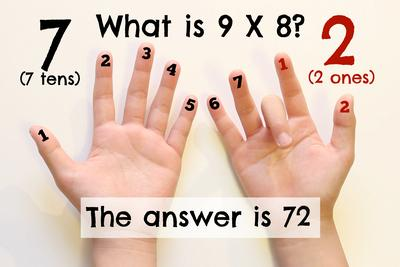Math Tricks! - Exceed in Learning

# Math Tricks!Isn’t it amazing how numbers work together?  Maybe you’ve seen some cool math tricks or possibly you know a few.  These math tricks are a quick and easy way to calculate numbers in your head, which will save you time!  Of course, it is important that your child understands the proper way to solve problems first.  Jumping to a “trick” right away is not the best thing to do.  For example, if your child is learning how to multiply, they need to understand the relationship with repeated addition, arrays, etc.  However, for children that understand these concepts, they may be ready for a few fun math tricks!  Let’s take a look at some that may be helpful for your child!

1. Multiplying a Two-Digit number x 11

We all know how to multiply by ten.  You just simply add a zero to the end of the other number, and voila!  But how about problems such as 78 x 11 or 45 x 11?  At first it may seem a little tricky but actually it is pretty simple!  Here is what you do.  Take a look at the number you are multiplying by.  For example, if you are multiplying by 45, add 4 + 5 together.  This number will go in between the 4 and 5.  Your answer would be 495.  Another example would be 62 x 11.  6 + 2 = 8 so the answer to 62 x 11 would be 682!  Simple enough!

But you may be wondering, what if I get a number such as 14 or 18 when I add the two numbers together?  How does this trick work?  It is a little different, but not much.  Let’s take a look at 78 x 11.  We first add 7 and 8 together (same as before), and we get 15.  Now, you add the number in the tens place with the first number.  (I know, this is completely opposite of what we usually do!)  The number in the ones place is your middle number.  Check out the graphic below for some help!1. Subtracting from 1,000

This trick works when you are subtracting a large number from 1,000.  Simply subtract the first two numbers from 9 and the last number from 10.  Here is an example.

1,000 – 384

Step 1: Subtract 3 from 9 = 6

Step 2: Subtract 8 from 9 = 1

Step 3: Subtract 4 from 10 = 6

1. Nines Trick

There are quite a few “nines tricks” for multiplication facts.  One trick is using your hands.  If you have the problem 9 x 8, count over 8 from the left side.  Put this finger down.  Count how many fingers are on the left side of the “down finger”.  Then count how many fingers are on the right side of the “down finger”.1. Comparing Fractions

There is a standard way to compare fractions.  You first have to find a common denominator and then make an equivalent fraction.  This can take a little bit of time, but thankfully there is an easier way.  Let me introduce the “Butterfly Trick” to you.To do this trick, draw circles around the diagonal numbers and multiply.  Write the product above each fraction.  Simple compare these numbers and that’s it!  This makes it pretty easy to mentally compare fractions.

1. Multiplying a Number by Five.

This works when you are using an even number times 5.  How this works is you divide the other number by two and add a zero at the end!  Let’s look at a few examples.  For the problem 86 x 5, divide 86 in half.  This equals 43.  Add a zero to the end and you have your answer, 430!

What are some other math tricks that you use?